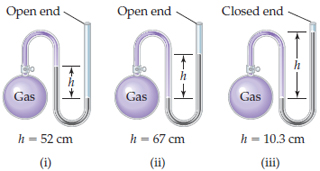# Problem: If the atmospheric pressure is 0.980 atm , what is the pressure of the enclosed gas in each of the three cases depicted in the drawing ?What is the pressure of the enclosed gas in (iii)? Assume that the gray liquid is mercury.

###### FREE Expert Solution

Step 1

= 103 mm

94% (233 ratings)###### Problem Details

If the atmospheric pressure is 0.980 atm , what is the pressure of the enclosed gas in each of the three cases depicted in the drawing?

What is the pressure of the enclosed gas in (iii)? Assume that the gray liquid is mercury.